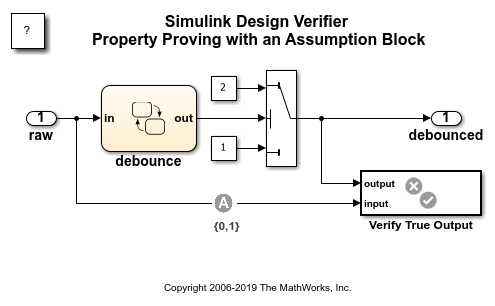# Property Proving with an Assumption Block

This example shows how to perform a Simulink Design Verifier property proof using a Proof Assumption block. It attempts to prove that when the sum of the current and six previous input values is greater than 6, the output equals 2. The model includes a Proof Assumption block that constrains the input to be 0 or 1. Simulink Design Verifier searches for violations of 20 or fewer time steps. It is unable to find a violation because the property is valid under the assumption.

```open_system('sldvdemo_debounce_assumeblk'); ```